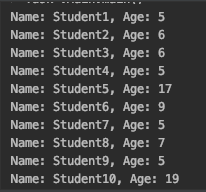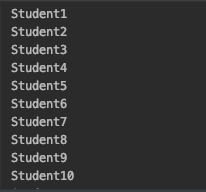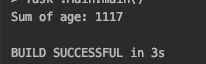## Introduction

Java stream API is not a really new API. This API has been introduced by Oracle since Java version 8. As specified inside the JDK documentation, Java stream is  A sequence of elements supporting sequential and parallel aggregate operations (source: https://docs.oracle.com/javase/8/docs/api/java/util/stream/Stream.html).

Stream API, when used properly, can help the use of collections simpler and easier, following the way of doing functional programming. Java stream API has plenty of operations available.

In today's post, we are going to run through some of the available operations provided by the stream API, including the sample source code and output from it. Due to so many operations available, we will limit our discussion to just several functions.

For the rest of the discussion, we will consider having TWO (2) classes, Student and ClassRoom. The code for the 2 classes is as below.
```public class Student {
private int age;
private String name;
public int getAge ( ) {
return age;    }

public void setAge ( int age ) {
this.age = age;    }

public String getName ( ) {
return name;    }

public void setName ( String name ) {
this.name = name;    }
}```

```public class ClassRoom {
private List< Student > students;
public List< Student > getStudents ( ) {
return students;    }

public void add ( Student student ) {
}```

To ensure we have data to play around with, let's add at least 100 students into the classroom. The code is as below.
```// Let's add 100 students to the ClassRoomClassRoom classRoom = new ClassRoom ( );
for ( int i = 0 ; i < 100 ; i++ ) {
int age = ( int ) ( Math.random ( ) * 20.0 + 1.0 );    String name = "Student" + ( i + 1 );
Student student = new Student ( );    student.setAge ( age );    student.setName ( name );

## ForEach

The forEach function performs an action for each element of this stream. This is a new way of doing repetition of action to be performed on each of the item in the stream. Mostly, we can use the forEach function instead of employing the traditional for construct.

Let's now try to print the name and age of all the students in the classroom. The code for this is pretty simple and straight forward.

```classRoom.getStudents ( ).stream ( ).forEach ( student ->
System.out.println ( "Name: " + student.getName ( ) + ", Age: " + student.getAge ( ) ) );```

Here is what happen with the above code:
• From the classRoom object, we call the getStudents function to retrieve the list of students under the class.
• From the list of students that we have, we call the stream function, to get the stream of data from the collection.
• From the stream data that we have, we call the forEach function.
One thing that might look strange for those who usually deal with older version of Java is the use of the -> operator. This operator is used to produce what is known as lambda expression.

What the expression does is, for each of an item in the students collection stream, we will give a name called student, and then we will perform an action which is defined after the -> operator (i.e.: System.out.println ( "Name: " + student.getName ( ) + ", Age: " + student.getAge ( ) )).

Below is the trimmed output of the above code. Note that when we add the student data, we are using random function, hence the age will be different in your case, and even in every execution.## Map

The map function returns a stream consisting of the results of applying the given function to the elements of this stream. This definition might sound abstract for those who are new to this concept. In short, the map function accepts the data and do transformation of the data based on the provided function. This transformed data then returned in a form of another stream.

Now, let's say, we want to extract the name of all the students and store it into a list. Here is how we can achieve it using the map and collect function.
```List studentNames = classRoom.getStudents ( ).stream ( )
.map ( student -> student.getName ( ) ).collect ( Collectors.toList ( ) );studentNames.stream ( ).forEach ( System.out::println );```

You can see inside the all of the map function, we assign the variable name student to each object inside the collection and then we tell the compiler to grab only the student's name by calling student.getName. After that, we call the collect function which will then collect the names of the students and convert it to a List object.

I add the printing of each of the students' name to the console by calling the forEach function, just like above, albeit with another variance.

Below is the trimmed output of the code above.## Sum

Now, let's say we want to get the sum of the age of all the students, we can utilise the sum function. This function though is not provided by the map directly. It is though provided by the LongStream, DoubleStream, and IntStream which can be produced by calling mapToLong, mapToDouble, and mapToInt respectively.

Now, for our example, we can use mapToInt function and then call the sum function in order to get the sum of all the ages. The code is as below.
```int sum = classRoom.getStudents ( ).stream ( )
.mapToInt ( student -> student.getAge ( ) ).sum ( );System.out.println ( "Sum of age: " + sum );```

Similar to the map function call, we tell the function mapToInt to return the stream of the students' age and then sum all the ages and return the value. Here is the output of the above code.## Filter

Filter is one of the most powerful functions provided by the stream API. Filter returns a stream consisting of the elements of this stream that match the given predicate.

Let's take a look on how can we grab the list of students whose age is between 15 and 17.

```classRoom.getStudents ( ).stream ( )
.filter ( student -> student.getAge ( ) >= 15 && student.getAge ( ) <= 17 )
.forEach ( student ->
System.out.println ( "Name: " + student.getName ( ) + ", Age: " + student.getAge ( ) ) );```

When we use the filter function, we feed in the condition of the values that we want. In our case, what we want is all students whose age is between 15 and 17, depicted by the code student.getAge ( ) >= 15 && student.getAge ( ) <= 17. Here is the output of the code.
`Name: Student8, Age: 15Name: Student10, Age: 17Name: Student26, Age: 15Name: Student33, Age: 15Name: Student36, Age: 15Name: Student47, Age: 17Name: Student51, Age: 17Name: Student57, Age: 17Name: Student64, Age: 16Name: Student67, Age: 17Name: Student74, Age: 16Name: Student84, Age: 16Name: Student95, Age: 16`

Again, note that since we randomise the age, the output can vary among different executions.

## Conclusion

As we can see, Java stream API provides useful functions we can use to make it easier to deal with collections. On all the example above, we can avoid the use of traditional for..loop.. construct and use the more functional approach to solve the problem and achieve what we want.

The code used for this blog is available at Github:
https://github.com/handracs2007/javastream

That's it for today, until next time.

1.This is really nice post, I love this content also visit Google Search Api. Thanks for sharing.